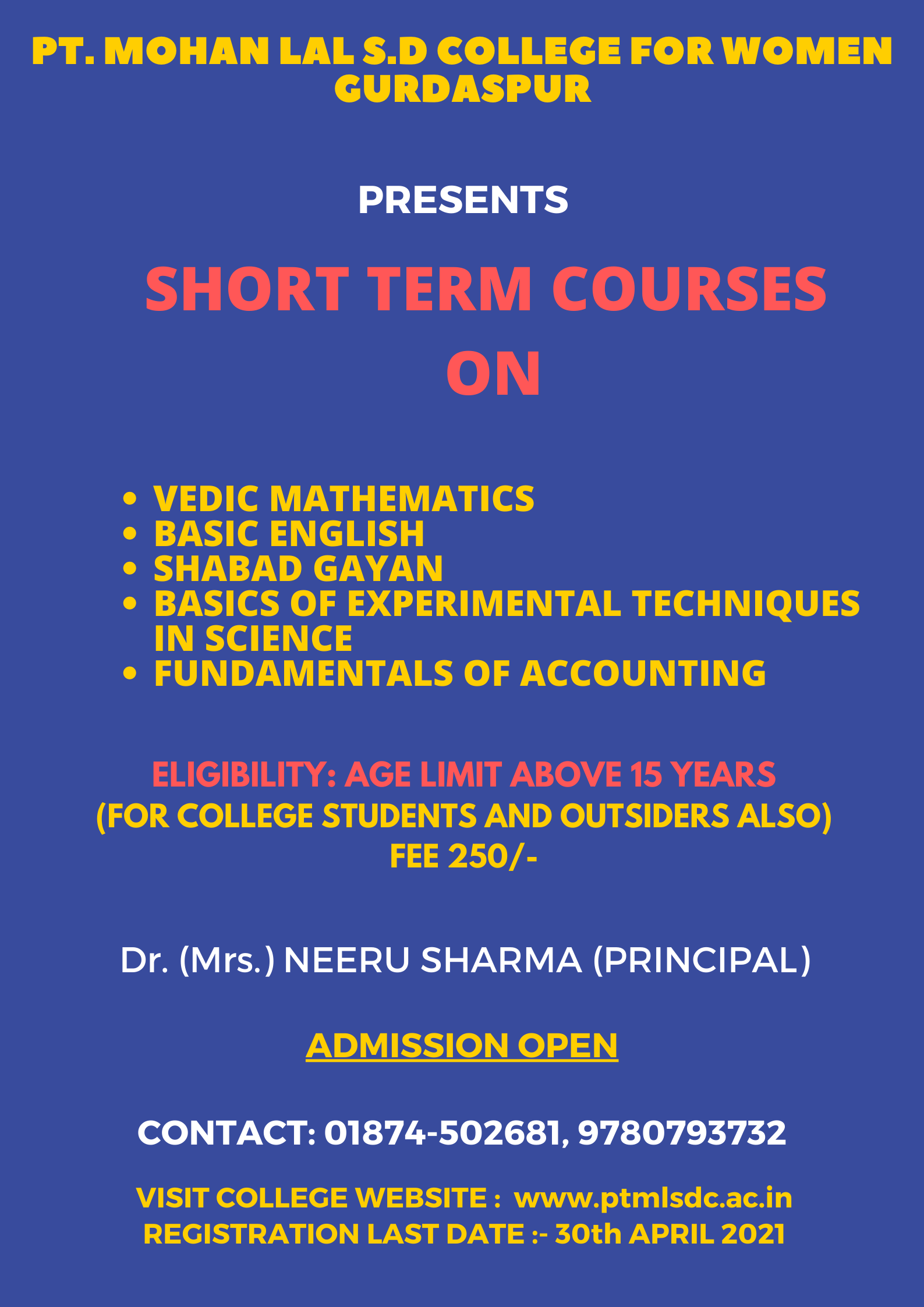VEDIC MATHVEDIC MATHEMATICS (OFFLINE)

The Department of Mathematics of Pt. Mohan Lal S.D. College for Women, Gurdaspur organized one month short term course on Vedic Mathematics from 7th June, 2021 to 7th July, 2021 for students. 20 students were enrolled for this course.

Vedic mathematics provides answer in one line where as conventional method requires several steps. It is an ancient technique which simplifies multiplication, divisibility, complex numbers, squaring cubing, square and cube roots. Even recurring decimals and auxiliary fractions can be handled by Vedic Mathematics.

The course instructor Ms. Diksha Malhotra covered all the topics under Vedic Mathematics and teaches students observational skills to do calculations faster. She gave hands on practice and elaborated on the following topics:-

v  One more than the previous one

o   All from 9 and last from 10

o   Addition - Completing the whole

o   Addition from left to right

o   Addition of list of numbers - Shudh method

o   Subtraction - Base method

o   Subtraction - Completing the whole

o   Subtraction from left to right

v   Criss-cross (Vertically and cross-wise)

v    Transpose and adjust (Transpose and apply)

o    If one is in ratio, the other one is zero

o    By addition and by subtraction

o     By the completion or non-completion

v  Base Method

o   Sub Base Method

o   Vinculum

o    Multiplication of complimentary numbers

o   Multiplication by numbers consisting of all 9s

o   Multiplication by 11

o   Multiplication by two-digit numbers from right to left

o   Multiplication by three and four-digit numbers from right to left

v  Squaring and square Roots

o   Squaring

o   Squaring numbers ending in 5

o   Squaring Decimals and Fraction Squaring Numbers Near 50

o   Squaring numbers near a Base and Sub Base

o   General method of Squaring - from left to right

o   Number splitting to simplify Squaring Calculation

o   Algebraic Squaring

v  Square Roots

o   Reverse squaring to find Square Root of Numbers ending in 25

o   Square root of perfect squares

o   General method of Square Roots

Vedic Mathematics is a collection of Techniques/Sutras to solve mathematical arithmetic’s in easy and faster way. It consists of 16 Sutras (Formulae) and 13 sub-sutras (Sub Formulae) which can be used for problems involved in arithmetic, algebra, geometry, calculus, conics.

In the latest trends Vedic math’s is rapidly developing and has attracted many students who are preparing for competitive exams. The Very Most Benefits of Vedic Math’s is It gives you the 10-15 times faster result as compared to the Western way of calculation. Vedic Mathematics Tricks is very useful in the aptitude section of the competitive exam. No need to remember any formula and dependency on the calculator will be less.

STUDENTS' FEEDBACK

Short Term Course was organized for Students of Pt. Mohan Lal S.D. College for Women, Gurdaspur. A good innovative workshop. It helped to think more. Good teaching helped more in knowing depthness of the maths as a subject.

Vedic Math’s has doubled their confidence and their ability to face different problems.

Pt. Mohan Lal S.D. College For Women